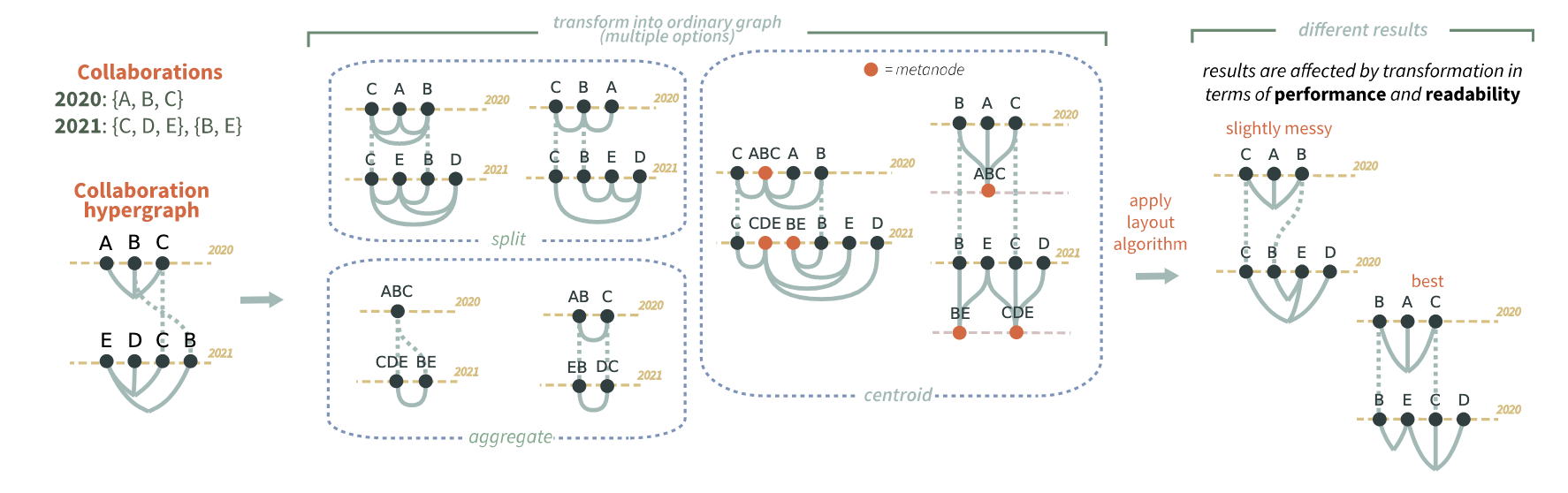### Six methods for transforming layered hypergraphs to apply layered graph layout algorithmsOn the left: Authors A, B, and C collaborate on a paper in 2020. In 2021, C works on another paper with D and E, while B works on a third paper with E. A collaboration network like this can be easily represented as a hypergraph where hyperedges denote co-authoring a paper. We show the network with a layered hypergraph visualization in which every layer corresponds to a year. In order to obtain a readable visualization, though, we need a layout algorithm, and to apply the algorithm to a hypergraph, we transform it into a graph. Yet, there are many methods for transforming a hypergraph into a layered graph, each with different layout readability and computational performance.
Abstract

Hypergraphs are a generalization of graphs in which edges (hyperedges) can connect more than two vertices—as opposed to ordinary graphs where edges involve only two vertices. Hypergraphs are a fairly common data structure but there is little consensus on how to visualize them. To optimize a hypergraph drawing for readability, we need a layout algorithm. Common graph layout algorithms only consider ordinary graphs and do not take hyperedges into account. We focus on layered hypergraphs, a particular class of hypergraphs that, like layered graphs, assigns every vertex to a layer, and the vertices in a layer are drawn aligned on a linear axis with the axes arranged in parallel. In this paper, we propose a general method to apply layered graph layout algorithms to layered hypergraphs. We introduce six different transformations for layered hypergraphs. The choice of transformation affects the subsequent graph layout algorithm in terms of computational performance and readability of the results. Thus, we perform a comparative evaluation of these transformations in terms of number of crossings, edge length, and impact on performance. We also provide two case studies showing how our transformations can be applied to real-life use cases. A copy of this paper with all appendices and supplemental material is available at osf.io/grvwu.

Materials
PDF | DOI | Supplement | BibTeX

Authors
#####Sara Di BartolomeoAlexis PisterPaolo BuonoCatherine PlaisantCody DunneJean-Daniel Fekete
Citation

Data Visualization @ Khoury — Northeastern University
West Village H, Room 302
440 Huntington Ave, Boston, MA 02115, USA